# HSSlive: Plus One & Plus Two Notes & Solutions for Kerala State Board

## BSEB Class 9 Maths Chapter 9 Areas of Parallelograms and Triangles Ex 9.3 Textbook Solutions PDF: Download Bihar Board STD 9th Maths Chapter 9 Areas of Parallelograms and Triangles Ex 9.3 Book AnswersBSEB Class 9 Maths Chapter 9 Areas of Parallelograms and Triangles Ex 9.3 Textbook Solutions PDF: Download Bihar Board STD 9th Maths Chapter 9 Areas of Parallelograms and Triangles Ex 9.3 Book Answers

BSEB Class 9th Maths Chapter 9 Areas of Parallelograms and Triangles Ex 9.3 Textbooks Solutions and answers for students are now available in pdf format. Bihar Board Class 9th Maths Chapter 9 Areas of Parallelograms and Triangles Ex 9.3 Book answers and solutions are one of the most important study materials for any student. The Bihar Board Class 9th Maths Chapter 9 Areas of Parallelograms and Triangles Ex 9.3 books are published by the Bihar Board Publishers. These Bihar Board Class 9th Maths Chapter 9 Areas of Parallelograms and Triangles Ex 9.3 textbooks are prepared by a group of expert faculty members. Students can download these BSEB STD 9th Maths Chapter 9 Areas of Parallelograms and Triangles Ex 9.3 book solutions pdf online from this page.

## Bihar Board Class 9th Maths Chapter 9 Areas of Parallelograms and Triangles Ex 9.3 Books Solutions

 Board BSEB Materials Textbook Solutions/Guide Format DOC/PDF Class 9th Subject Maths Chapter 9 Areas of Parallelograms and Triangles Ex 9.3 Chapters All Provider Hsslive

## How to download Bihar Board Class 9th Maths Chapter 9 Areas of Parallelograms and Triangles Ex 9.3 Textbook Solutions Answers PDF Online?

2. Click on the Bihar Board Class 9th Maths Chapter 9 Areas of Parallelograms and Triangles Ex 9.3 Answers.
3. Look for your Bihar Board STD 9th Maths Chapter 9 Areas of Parallelograms and Triangles Ex 9.3 Textbooks PDF.
4. Now download or read the Bihar Board Class 9th Maths Chapter 9 Areas of Parallelograms and Triangles Ex 9.3 Textbook Solutions for PDF Free.

## BSEB Class 9th Maths Chapter 9 Areas of Parallelograms and Triangles Ex 9.3 Textbooks Solutions with Answer PDF Download

Find below the list of all BSEB Class 9th Maths Chapter 9 Areas of Parallelograms and Triangles Ex 9.3 Textbook Solutions for PDF’s for you to download and prepare for the upcoming exams:

## BSEB Bihar Board Class 9th Maths Solutions Chapter 9 Areas of Parallelograms and Triangles Ex 9.3

Question 1.
In figure, E is any point on median AD of a ∆ ABC. Show that ar(ABE) = ar(ACE).Solution:
Given : AD is a median of ∆ ABC and E is any point on AD.
To prove : ar(ABE) = ar(ACE).
Proof:
∵ AD is the median of ∆ ABC
∴ ar(ABD) = ar(ACD) … (1)
Also, ED is the median of ∆ EBC
∴ ar(BED) = ar(CED) … (2)
Subtracting (2) from (1), we get
ar(ABD) – ar(BED) = ar(ACD) – ar(CED)
⇒ ar(ABE) = ar(ACE).

Question 2.
In a triangle ABC, E is the mid-point of median AD. Show that ar(BED) = 14 ar(ABC).
Solution:
Given : ∆ ABC, E is the mid-point of the median AD.
To prove : ar(BED) = 14 ar(ABC).Proof : Since AD is a median of ∆ ABC and median divides a triangle into two triangles of equal area.
⇒ ar(ABD) = 12 ar(ABC) … (1)
In ∆ ABD, BE is the median
∴ ar(BED) = 12 ar(BAE)
⇒ ar(BED) = 12 ar(ABD) … (2)
⇒ ar(BED) = 12 x 12ar(ABC) [Using (1)]
⇒ ar(BED) = 12 ar(ABC).

Question 3.
Show that the diagonals of a parallelogram divide it into four triangles of equal area.
Solution:
Given : A parallelogram ABCD.
To prove : The diagonals AC and BD divide the ||gm ABCD into four triangles of equal area.
Construction : Draw BL ⊥ AC.
Proof : Since ABCD is a ||gm and so its diagonals AC and BD bisect each other at O.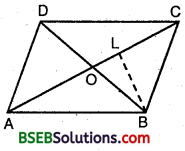∴ AO = OC and BO = OD
Now, ar(AOB) = 12 x AO x BL
ar(OBC) = 12 x OC x BL
But AO = OC
∴ ar(AOB) = ar(OBC)
Similarly, we can show that
ar(OBC) = ar(OCD);
ar(OCD) = ar(ODA);
ar(ODA) = ar(OAB);
ar(OAB) = ar(OBC)
ar(OCD) = ar(ODA)
Thus, ar(OAB) = ar(OBC) = ar(OCD) = ar(OAD).

Question 4.
In figure, ABC and ABD are two triangles on the same base AB. If line segment CD is bisected by AB at O, show that ar(ABC) = ar(ABD).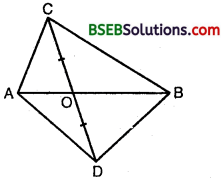Solution:
Given : ABC and ABD are two triangles on the same base AB. A line segment CD is bisected by AB at O i.e., OC = OD.
To prove : ar(ABC) = ar(ABD).
Proof : In ∆ ACD, we have
OC = OD [Given]
∴ AO is the median.
ar(AOC) = ar(AOD) …(1)
[∵ Median divides a ∆ in two As of equal area]
Similarly, in ∆ BCD, BO is the median
∴ ar(BOC) = ar(BOD) … (2)
Adding (1) and (2), we get
ar(AOC) + ar(BOC) = ar(AOD) + ar(BOD)
⇒ ar(ABC) = ar(ABD).

Question 5.
D, E and F are respectively the mid-points of the sides BC, CA and AB of a ∆ ABC. Show that
(i) BDEF is a parallelogram
(ii) ar(DEF) = 14 ar(ABC)
(iii) ar(BDEF) = 14 ar(ABC).
Given : D, E and F are the mid-points of the sides BC, CA and AB respectively of ∆ ABC.
To prove : (i) BDEF is a ||gm
(ii) ar(DEF) = 14 ar(ABC)
(iii) ar(BDEF) = 12 ar(ABC)
Solution:
(i) We have in ∆ ABC,
EF || BC [By mid-point theorem, since E and F are the mid-points of AC and AB respectively]
∴ EF || BD … (1)
Also, ED || AB [By mid-point theorem, since E and D are the mid-points of AC and BC respectively]
∴ ED || AF
From (1) and (2), BDEF is a ||gm.

(ii) Similarly, FDCE and AFDE are ||gms.
∴ ar(FBD) = ar(DEF) [∵ FD is a diagonal of ||gm BDEF]
ar(DEC) = ar(DEF) [∵ ED is a diagonal of ||gm FDCE]
and, ar(AFE) = ar(DEF) [∵ FE is a diagonal of ||gm AFDE]
∴ ar(FBD) = ar(DEC) = ar(AFE) = ar(DEF) …(3)
⇒ ar(DEF) = 14 ar(ABC).

(iii) Also, ar(BDEF) = 2 ar(DEF)
= 2 x 14 ar(ABC) = 12ar(ABC).

Question 6.
In figure, diagonals AC and BD of quadrilateral ABCD interest at O such that OB = OD. If AB = CD, then show that: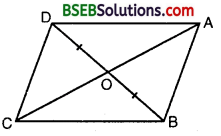(i) ar(DOC) = ar(AOB)
(ii) ar(DCB) = ar(ACB) C
(iii) DA || CB or ABCD is a parallelogram.
Solution:
(i) Draw DN ⊥ AC and BM ⊥ AC.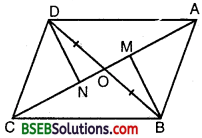In ∆s DON and BOM
∠DNO = ∠BMO [Each = 90°)
∠DON = ∠BOM [Vert. opp. ∠s]
OD = OB [Given]
By AAS criterion of congruent Q
∆ DON ≅ ∆ BOM … (1)
In ∆s DCN and PAM
∠DNC = ∠BMA [Each = 90°]
DC = AB [Given]
DN = BM [∵ A DON ≅ A BOM ⇒ DN = BM]
∴ By RHS criterion of congruence,
∆ DCN ≅ ∆ BAM
From (1) and (2), we get
ar(DON) + ar(DCN) = ar(BOM) + ar(BAM)
⇒ ar(DOC) = ar(AOB).
(ii) Since ar(DOC) = ar(AOB)
∴ ar(DOC) + ar{BOC) = ar(AOB) + ar(BOC)
⇒ ar(DCB) = ar(ACB).

(iii) ∆s DCB and ACB have equal areas and have the same base. So, these As lie between the same parallels.
⇒ DA || CB i.e., ABCD is a ||gm.

Question 7.
D and E are points on sides AB and AC respectively of ∆ ABC such that ar(DBC) = ar(EBC) Prove that DE || BC.
Solution:
Since As DBC and EBC are equal in area and have a same base BC.∴ Altitude from D of ∆ DBC = Altitude from E of ∆ EBC.
⇒ As DBC and EBC are between the same parallels.
⇒ DE || BC.

Question 8.
XY is a line parallel to side BC of a triangle ABC. If BE || AC and CF || AB meet XY at E and F respectively, show that ar(ABE) = ar(ACF).
Solution:
Since XY || BC and BE || CY.
∴ BCYE is a ||gm.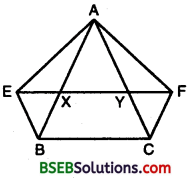Since ∆ ABE and ||gm BCYE are on the same base BE and between the same parallel lines BE and AC.
∴ ar(ABE) = 12ar(BCYE) …(1)
Now, CF || AB and XY || BC
⇒ CF || AB and XF || BC
⇒ BCFX is a ||gm.
Since ∆ ACF and ||gm BCFX are on the same base CF and between the same parallel AB and FC
∴ ar(ACF) = 12ar(BCFX) …(2)
But ||gm BCFX and ||gm BCYE are on the same base BC and between the same parallels BC and EF.
∴ ar(BCFX) = ar(BCYE) … (3)
From (1), (2) and (3), we got
∴ ar(∆ ABE) = ar(∆ ACF).

Question 9.
The side AB of a parallelogram ABCD is produced to any point P: A line through A and parallel to CP meets CB produced at Q and then parallelogram PBQR is completed (see figure). Show that ar(ABCD) = ar(PBQR).Solution:
Join AC and PQ. Since AC and PQ are diagonals of ||gm ABCD and ||gm BPQR respectively.∴ ar(ABC) = 12 ar(ABCD)… (1)
and, ar(PBQ) = 12 ar(BPRQ) … (2)
Now, ∆s ACQ and AQP are on the same base AQ and between the same parallels AQ and CP.
∴ ar(ACQ) = ar(AQP)
⇒ ar(ACQ) – ar(ABQ) = ar(AQP) – ar(ABQ) [Subtracting ar(ABQ) from both sides]
⇒ ar(ABC) = ar(BPQ)
⇒ 12 ar(ABCD) = 12 ar(BPRQ) [Using (1) and (2)]
⇒ ar(ABCD) = ar(BPRQ).

Question 10.
Diagonals AC and BD of a trapezium ABCD with AB || DC intersect each other at O. Prove that ar(AOD) = ar(BOC).
Solution:
Diagonals AC and BD of a trapezium ABCD with AB || DC intersect each other at O.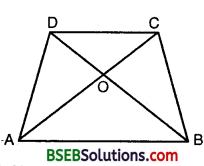∴ ∆s ABC and ABD are on the same base and between the same parallels. A
∴ ar(ABD) = ar(ABC)
⇒ ar(ABD) – ar(AOB) = ar(ABC) – ar(AOB) [Subtracting ar(AOB) from both sides]
⇒ ar(AOD) = ar(BOC).

Question 11.
In figure, ABCDE is A B a pentagon. A line through B parallel to AC meets DC produced at F. Show that
(i) ar(ACB) = ar(ACF)
(ii) ar(AEDF) = ar(ABCDE).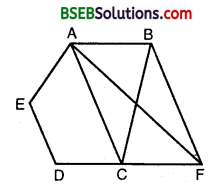Solution:
(i) Since ∆s ACB and ACF are on the same base AC and between the same parallels AC and BF.
∴ ar(ACB) = ar(ACF).

(ii) Adding ar(ACDE) on both sides, we get
ar(ACF) + ar(ACDE) = ar(ACB) + ar(ACDE)
⇒ ar(AEDF) = ar(ABCDE).

Question 12.
A villager Itwaari has a plot of land of the shape of a quadrilateral. The Gram Panchayat of the village decided to take over some portion of his plot from one of the corners to construct a Health Centre. Itwaari agrees to the above proposal with the condition that he should be given equal amount of land in lieu of his land adjoining his plot so as to form a triangular plot. Explain how this proposal will be implemented.
Solution:
Let ABCD be the quadrilateral plot. Produce BA to meet DE drawn parallel to CA at E. Join EC.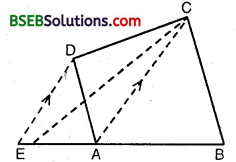Then, ∆s EAC and ADC lie on the same parallels DE and CA
Now, ar(ABCD) = ar(ABC) + ar(ACD)
= ar(ABC) + ar(EAC) = ar(EBC)
i. e., quad. ABCD = ∆ EBC
which is the required explain to the suggested proposal.

Question 13.
ABCD is a trapezium with AB || DC. A line parallel to AC intersects AB at X and BC at Y. Prove that ar(ADX) = ar(ACY).
Solution:
ABCD is a trapezium with AB || DC and XY || AC, is drawn. Join XC.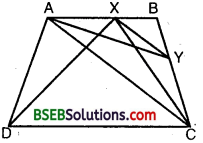ar(ACX) = ar(ACY) … (1) [∵ As ACX and ACY have same base AC and are between same parallels AC and XY]
But ar(ACX) = ar(ADX) … (2) [∵ As ACX and ADX have same base AX and are between same parallels AB and DC]
From (1) and (2), we have ar(ADX) = ar(ACY).

Question 14.
In figure, AP || BQ || CR. Prove that
ar(AQC) = ar(PBR).Solution:
From the figure, we have
ar(AQC) = ar(AQB) + ar(BQC) …(1)
and, ar(PBR) = ar(PBQ) + ar(QBR) …(2)
But ar(AQB) = ar(PBQ) …(3)
[∵ These As are on the same base BQ and between same parallel lines AP and BQ]
Also, ar(BQC) = ar(QBR) …(4)
[∵ These As are on the same base BQ and between same parallel lines BQ and CR]
Using (3) and (4) in (1) and (2), we get
ar(AQC) = ar(PBR).

Question 15.
Diagonals AC and BD of a quadrilateral ABCD intersect at O in such a way that ar(AOD) = ar(BOC). Prove that ABCD is a trapezium.
Solution:
Diagonals AC and BD of a quadrilateral ABCD intersect at O in such a way that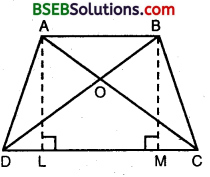ar(AOD) = ar(BOC) … (1)
Adding ar (ODC) on both sides, we get
ar(AOD) + ar(ODC) = ar(BOC) + ar(ODC)
⇒ 12 x DC x AL = 12 x DC x BM
⇒ AL = BM
⇒ AB || DC
Hence, ABCD is a trapezium.

Question 16.
In figure, ar(DRC) = ar(DPC) and ar(BDP) = ar(ARC). Show that both the quadrilaterals ABCD and DCPR are trapeziums.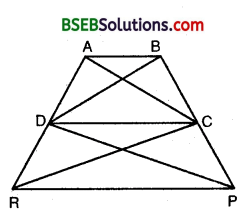Solution:
From the figure,
ar(BDP) = ar(ARC) [Given]
and ar(DPC) = ar(DRC) [Given]
On subtracting, we get
ar(BDP) – ar(DPC) = ar(ARC) – ar(DRC)
⇒ DC || AB
Hence, ABCD is a trapezium.
ar(DRC) = ar(DPC) [Given]
On subtracting ar(DLC) from both sides, we get
ar(DRC) – ar(DLC) = ar(DPC) – ar(DLC)
⇒ ar(DLR) = ar(CLP)
On adding ar(RLP) to both sides, we get
ar(DLR) + ar(RLP) = ar(CLP) + ar(RLP)
⇒ ar(DRP) = ar(CRP)
⇒ RP || DC
Hence, DCPR is a trapezium.

## Bihar Board Class 9th Maths Chapter 9 Areas of Parallelograms and Triangles Ex 9.3 Textbooks for Exam Preparations

Bihar Board Class 9th Maths Chapter 9 Areas of Parallelograms and Triangles Ex 9.3 Textbook Solutions can be of great help in your Bihar Board Class 9th Maths Chapter 9 Areas of Parallelograms and Triangles Ex 9.3 exam preparation. The BSEB STD 9th Maths Chapter 9 Areas of Parallelograms and Triangles Ex 9.3 Textbooks study material, used with the English medium textbooks, can help you complete the entire Class 9th Maths Chapter 9 Areas of Parallelograms and Triangles Ex 9.3 Books State Board syllabus with maximum efficiency.

## FAQs Regarding Bihar Board Class 9th Maths Chapter 9 Areas of Parallelograms and Triangles Ex 9.3 Textbook Solutions

#### How to get BSEB Class 9th Maths Chapter 9 Areas of Parallelograms and Triangles Ex 9.3 Textbook Answers??

Students can download the Bihar Board Class 9 Maths Chapter 9 Areas of Parallelograms and Triangles Ex 9.3 Answers PDF from the links provided above.

#### Can we get a Bihar Board Book PDF for all Classes?

Yes you can get Bihar Board Text Book PDF for all classes using the links provided in the above article.

## Important Terms

Bihar Board Class 9th Maths Chapter 9 Areas of Parallelograms and Triangles Ex 9.3, BSEB Class 9th Maths Chapter 9 Areas of Parallelograms and Triangles Ex 9.3 Textbooks, Bihar Board Class 9th Maths Chapter 9 Areas of Parallelograms and Triangles Ex 9.3, Bihar Board Class 9th Maths Chapter 9 Areas of Parallelograms and Triangles Ex 9.3 Textbook solutions, BSEB Class 9th Maths Chapter 9 Areas of Parallelograms and Triangles Ex 9.3 Textbooks Solutions, Bihar Board STD 9th Maths Chapter 9 Areas of Parallelograms and Triangles Ex 9.3, BSEB STD 9th Maths Chapter 9 Areas of Parallelograms and Triangles Ex 9.3 Textbooks, Bihar Board STD 9th Maths Chapter 9 Areas of Parallelograms and Triangles Ex 9.3, Bihar Board STD 9th Maths Chapter 9 Areas of Parallelograms and Triangles Ex 9.3 Textbook solutions, BSEB STD 9th Maths Chapter 9 Areas of Parallelograms and Triangles Ex 9.3 Textbooks Solutions,
Share: